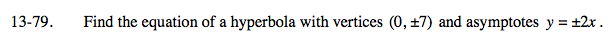### Home > A2C > Chapter 13 > Lesson 13.1.4 > Problem13-79

13-79.The center will be at (0, 0).

$\text{In the equation }\frac{y^2}{A}-\frac{x^2}{B}=1$

$\sqrt{A}=2\sqrt{B}$

$\frac{y^2}{49}-\frac{x^2}{12.25}=1$